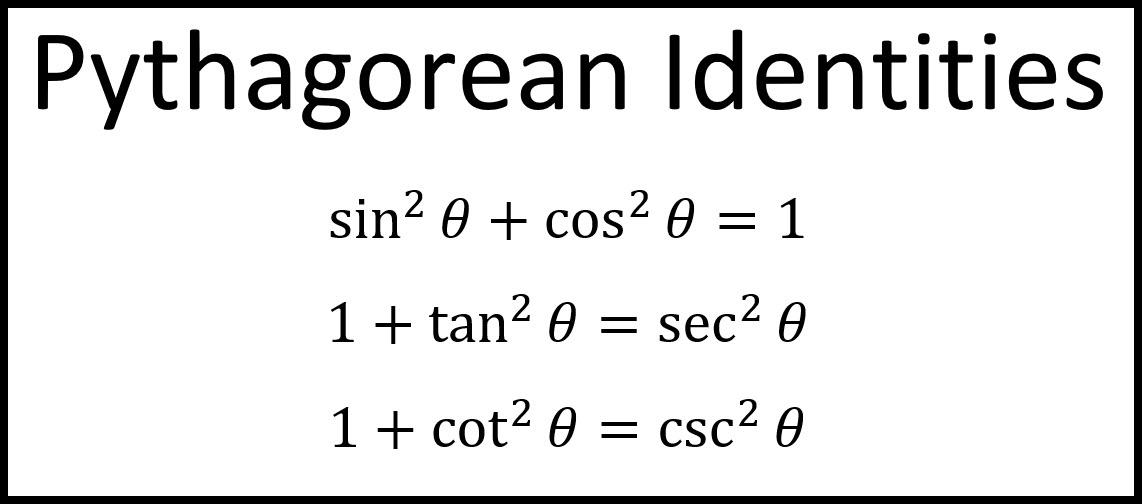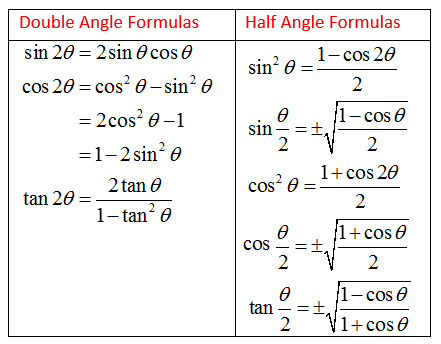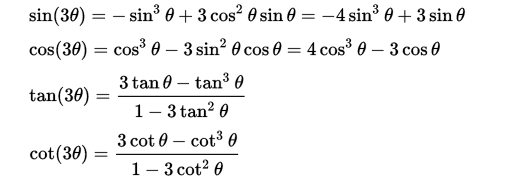# List of Trigonometry Formulas and Identities

Trigonometry Formula: Trigonometry is the mathematical branch that deals with the relationship between triangle’s angles and sides. There are many intriguing applications of trigonometry which can be used in everyday life.

For instance, if you are sitting on the balcony of a tall structure that has a certain height and notice a post box across the street, then you are able to quickly determine the distance of the road by using trigonometry.

Book A Free Demo# Application use of Trigonometry Formulas

These formulas can be useful in astronomy since they are able to determine the distance between stars and satellites. Construction as well as flight engineering, criminology, marine biology, engineering, as well as many other fields use trigonometry formulae.

High school students are typically taught the basics of trigonometry in Class 9 or Class 10. . 10th grade Trigonometry Formula can be used to determine the sides of different angles. The formulas are categorized into different categories based on the application .As we proceed to Classes 11 as well as Class 12 teachers cover more advanced concepts related to trigonometry. Students might be overwhelmed due to the various concepts that are covered. To help you, we’ve made a complete collection of trigonometry formulas for classes 10,11 and 12.

To find out more,we’ve provided a listing of all trigonometry formulas along with the ratio table, to help them learn to determine angles’ sides as well as calculate trigonometry numbers and calculate the distance between two landmarks.

## List of Trigonometry Formulas

When studying trigonometric equations, we must consider only right-angled triangles. The Trigonometry Formula focuses on other triangles.

In a right-angled triangle, you will find three edges: the hypotenuse and the other side (perpendicular) as well as the side adjacent (base). The longest of the sides is called the hypotenuse. The side that is opposite from the angle is known as the perpendicular side, and the side on which both the hypotenuse and the opposite side meet is the side adjacent to it.## What are the 11 trigonometric identities?

A variety of sets of formulas used in trigonometry are listed below:

1. Basic Formulas
2. Reciprocal Identities
3. Trigonometric Ratio Table
4. Periodic Identities
5. Cofunction Identities
6. Sum and Difference of Identities
7. Half-Angle Identities
8. Double Angle Identities
9. Triple Angle Identities
10. Product Identities
11. Sum of Product Identities

Book A Free Demo

## What are the basic formulas of trigonometry?

There are six essential trigonometric ratios that are utilized in all trigonometry formulas. The ratios can also be referred to as trigonometric functions , and mainly utilize all trigonometry equations. The six fundamental trigonometric functions include Sine cosine, Secant, cosecant, cotangent and tangent. The trigonometric identities and functions are determined by making use of the right-angled triangular. If the height and base of the right-angled triangle is identified we can determine the sine cosine, tangent Secant cosecant, secant, and cotangent values by using trigonometric formulas

In Right Triangle ABC

sinθ = side opposite to angle θ

—————————-——

hypotenuse

cos θ = side adjacent to angle θ

—————————–—

Hypotenuse

tan θ = side opposite to angle θ

———————————

cosec θ = 1          Hypotenuse

——— = ——————

sin θ    side opposite to angle θ

secθ  = 1           hypotenuse

——– = ———————

cos θ        side adjacent to the angle θ

cot θ = 1         side adjacent to the angle θ

——- = —————————————

tan  θ       side opposite to angle θ

Book A Free Demo

## What are six Reciprocal Identities of Trigonometry?

Reciprocal Identities

sin (θ) = 1                         csc (θ) = 1

————                              ———

csc (θ)                               sin (θ)

cos (θ) = 1                        sec θ= 1

——-                                   ——-

sec (θ)                               cos (θ)

tan (θ) = 1                         cot (θ) = 1

——                                      ——-

cot (θ)                                tan (θ)

Book A Free Demo

## What are three Pythagorean identities?## Trigonometric Ratios Table

The below trigonometry table will help you to find the values of standard trigonometric angles.## Cofunction Identities (in Degrees)## Sum and Difference of Identities

sin(a+b) = sin a cos b + cos a sin b

sin(a -b) = sin a cos b – cos a sin b

cos(a+b) = cos a cos b – sin a sin b

cos(a-b) = cos a cos b + sin a sin b

tan (a+b) = tan a + tan b

——————–

1 – tana tanb

tan (a-b) = tana – tan b

——————

1 + tana tanb

## Half Angle Identities and Double Angle IdentitiesBook A Free Demo

## Triple Angle Identities## Product Identities

sin(x)cos(y) = 1

—  [sin(x+y) + sin (x-y)]

2

cos(x)sin(y) = 1

—-[sin(x+y) – sin (x-y)]

2

cos(x)cos(y) = 1

— [cos(x-y) + cos(x+y)]

2

sin(x)sin(y) = 1

— [cos(x-y) – cos (x+y)]

2

## Sum of Product Identities

The combination of two of the acute angles A and B, can be represented through the trigonometric ratios, as shown below:

sin(x)cos(y) = 1

—  [sin(x+y) + sin (x-y)]

2

cos(x)sin(y) = 1

— [sin(x+y) – sin (x-y)]

2

cos(x)cos(y) = 1

— [cos(x-y) + cos(x+y)]

2

sin(x)sin(y) = 1

— [cos(x-y) – cos (x+y)]

2

Book A Free Demo

## How to remember trigonometry formulas?There are many formulas that we have in the higher classes that may make it difficult for us to recall, therefore there are steps to follow to remember the following:

2. Then comes the structure of formulas and the way they are calculated.
3. Do the exercises regularly.
4. Make flashcards of the formulas, review and then test your knowledge.

Book A Free Demo

## Master Trigonometry from the best Online Math’s tutors

With Guru At Home, you will be able to learn trigonometry formulas for classes 10, 11, and 12 and also solve trigonometry-related questions effectively. Guru At Home presents students with an amazing opportunity. Book a free counseling session with our math expert to master trigonometry. We have the best online math tutors with experience ranging from 1 year to 10+ years.

In the above article we have discussed trigonometry formulas, such as fundamental formulas, reciprocal identities, trigonometric ratio table periodic identity, cofunction identity, sums and difference identities half-angle identities, double angle identities, as well as triple-angle product identities as well as their sum identity. We talked about how to master the trigonometry formulas.

We hope that you will enjoy them and find them helpful. Learn to prepare for your exam by using similar methods. If you feel we’ve missed something or have any suggestions, please inform us. We would love to share your thoughts, and we will update our article in order to provide more value. Guru At Home wishes you the best for your studies.

Book A Free Demo

Scroll to Top